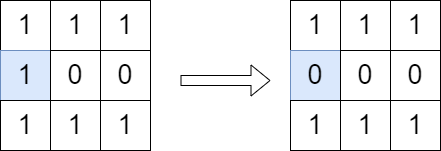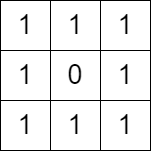2556. Disconnect Path in a Binary Matrix by at Most One Flip
Medium
364
13

You are given a 0-indexed `m x n` binary matrix `grid`. You can move from a cell `(row, col)` to any of the cells `(row + 1, col)` or `(row, col + 1)` that has the value `1`. The matrix is disconnected if there is no path from `(0, 0)` to `(m - 1, n - 1)`.

You can flip the value of at most one (possibly none) cell. You cannot flip the cells `(0, 0)` and `(m - 1, n - 1)`.

Return `true` if it is possible to make the matrix disconnect or `false` otherwise.

Note that flipping a cell changes its value from `0` to `1` or from `1` to `0`.

Example 1:```Input: grid = [[1,1,1],[1,0,0],[1,1,1]]
Output: true
Explanation: We can change the cell shown in the diagram above. There is no path from (0, 0) to (2, 2) in the resulting grid.
```

Example 2:```Input: grid = [[1,1,1],[1,0,1],[1,1,1]]
Output: false
Explanation: It is not possible to change at most one cell such that there is not path from (0, 0) to (2, 2).
```

Constraints:

• `m == grid.length`
• `n == grid[i].length`
• `1 <= m, n <= 1000`
• `1 <= m * n <= 105`
• `grid[i][j]` is either `0` or `1`.
• `grid == grid[m - 1][n - 1] == 1`
Accepted
8.4K
Submissions
29.6K
Acceptance Rate
28.4%

Seen this question in a real interview before?
1/4
Yes
No

Discussion (0)

Related Topics